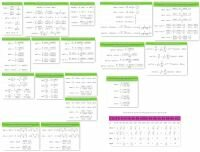Trigonometric

Trigonometric formulas

Trigonometric formulas or trigonometric identities describe the relationships between sine, cosine, tangent and cotangent and are used in solving mathematical problems.

The following are double angle formula, values of trigonometric functions, half angle formula, double angle identities, and other formulas. Additionally, the values of trigonometric functions for the most common angles are given.

Basic identities

... preparation ...

Double-angle formulas

... preparation ...

Three-angle formulas

... preparation ...

Formulas for reducing the degree

... preparation ...

Formulas for reducing the degree

... preparation ...

Formulas for reducing the degree

... preparation ...

The reduction formulas of the degree of the half argument

... preparation ...

... preparation ...

The subtraction formulas

... preparation ...

The formulas for converting a sum into product formulas

... preparation ...

The formulas for converting the difference into product formulas

... preparation ...

The sum conversion formulas

... preparation ...

The formulas for transforming a product into sum and difference formulas

... preparation ...

Formulas for transforming a product of functions to a power

... preparation ...

Formulas for reducing the degree

... preparation ...

Universal trigonometric substitution

... preparation ...

The values of trigonometric functions

 α 0 α° 0° 30° 45° 60° 90° 120° 135° 150° 180° 210° 225° 240° 270° 300° 315° 330° 360° sin α 0 1 0 −1 0 cos α 1 0 −1 0 1 tg α 0 1 − −1 0 1 − −1 0 ctg α − 1 0 −1 − 1 0 −1 −